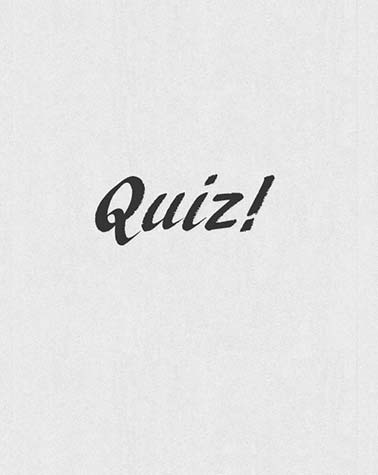# Infinity - An Online Quiz

13 Questions | Total Attempts: 59SettingsPrastuti'19 The Annual Technical fest of Electrical Dept, IIT BHU presents you with an exciting quiz competition based on logical reasoning and mathematical puzzles. Be ready to solve the puzzles and get prizes.

• 1.
ON and OFF -  There are 80 bulbs, all switched OFF. All the bulbs are switching ON and OFF to form a pattern. In the first round, every bulb is switched ON one by one. In the second round, every even-numbered bulb is switched OFF starting from 2nd, 4th, 6th,... In the third round, 3rd, 6th, 9th….bulbs are altered that is switching ON if they were OFF and switching OFF if they were ON. And so on.. A total of 80 such rounds are completed. After the completion of all rounds, what is the state of all the bulbs? Total [Blank] bulbs are in ON state.
• 2.
Can you find prime or not?? Find whether the number 1600040001 is prime or composite?? (Without using a calculator or computer) Hint: Ex. 8999 = 90000-1 = x2 -1 (where x is 300)
• A.

Prime

• B.

Composite

• 3.
Which of these numbers is known as Ramanujan Number?
• A.

1829

• B.

1729

• C.

1789

• D.

1879

• 4.
Fastest 3 athletes?? You are a coach of 25 athletes and you have to choose the fastest 3 from them to nominate for an international race. There are 5 lanes on a race track. The minimum number of races to be conducted in order to determine the fastest 3 racers is [Blank].
• 5.
Next Number?   The next number in the sequence  31, 29, 31, 30, [Blank]
• 6.
In a country where everyone wants a boy, each family continues having babies until they have a boy. After some time, the proportion of boys to girls in the country is [Blank] . (Assuming probability of having a boy or a girl is the same)
• 7.
Let p be a prime number, and a be any integer. Then ap - a is always divisible by p. This fundamental theorem of number theory is called
• A.

Fermat's Little theorem

• B.

Chinese Remainder Theorem

• C.

Lucas's Theorem

• D.

Euler's Theorem

• 8.
Let  or phi(n) be Euler's totient function. If  is a positive integer,  is the number of integers in the range  which are relatively prime to .  Let n  = p*q where p and q are prime numbers, Then
• A.

Phi(n) = p*q

• B.

Phi(n) = phi(p)*phi(q)

• C.

Phi(n) = n-1

• D.

Phi(n) = p*q - 1

• 9.
What is the remainder obtained when 270 + 370 is divided by 13
• A.

1

• B.

2

• C.

3

• D.

0

• 10.
The last two digits in 7979 are [Blank].
• 11.
The number of trailing zeros in 30! is [Blank].
• 12.
There is an earthquake which is one point higher on the Richter scale than another earthquake which is ten times powerful. Now how much powerful do you think the earthquake will be if it was just 1/2 a point higher on the Richter scale?
• A.

5 times

• B.

100 times

• C.

3.16 times

• D.

Double

• 13.
GCD of the following numbers is
• A.

728

• B.

2186

• C.

242

• D.

80Back to top